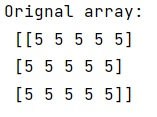# NumPy array initialization (fill with identical values)

In this tutorial, we will learn how to initialize a NumPy array and fill with identical values? By Pranit Sharma Last updated : May 23, 2023

NumPy is an abbreviated form of Numerical Python. It is used for different types of scientific operations in python. Numpy is a vast library in python which is used for almost every kind of scientific or mathematical operation. It is itself an array which is a collection of various methods and functions for processing the arrays.

Suppose we need to create a NumPy array of length n, and each element of this array would be e a single value (say 5).

## NumPy Array Initialization

To initialize a NumPy array and fill with identical values, you can use a method provided by NumPy called the full() method. This method is better than the empty() followed by the fill() method.

This is arguably the way of creating an array filled with certain values because it explicitly describes what is being achieved.

Let us understand with the help of an example,

## Python program to initialize a NumPy array and fill with identical values

```# Import numpy
import numpy as np

# Creating a numpy array
# using full() method
arr = np.full((3, 5), 5)

# Display original array
print("Orignal array:\n",arr,"\n")
```

### Output